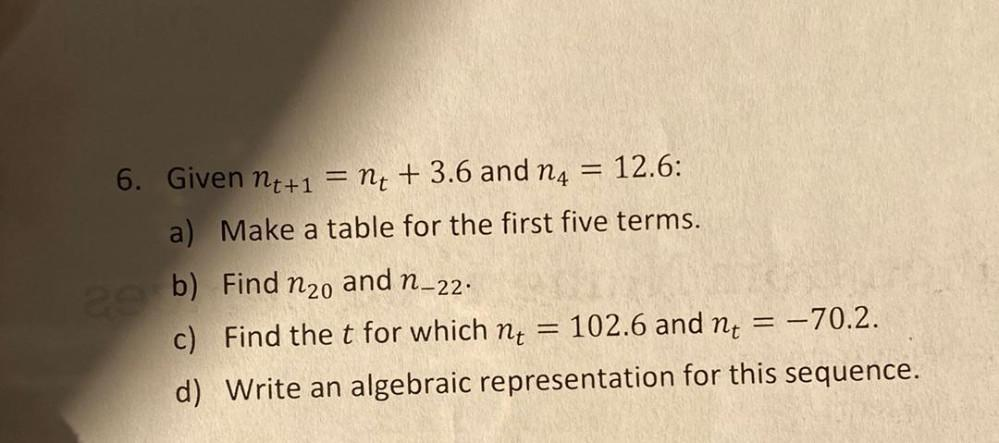Question:

# 6. Given nt+1 = nt + 3.6 and 14 = 12.6: a) Make a table for the first five terms. b) Find n2o and n–22. c) Find the t for which6. Given nt+1 = nt + 3.6 and 14 = 12.6: a) Make a table for the first five terms. b) Find n2o and n–22. c) Find the t for which nt 102.6 and nt = = -70.2. d) Write an algebraic representation for this sequence. ==